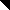# MINLPLib

### A Library of Mixed-Integer and Continuous Nonlinear Programming Instances

#### Instance nvs09

 Formatsⓘ ams gms mod nl osil py Primal Bounds (infeas ≤ 1e-08)ⓘ -43.13433692 p1 ( gdx sol ) (infeas: 0) Other points (infeas > 1e-08)ⓘ Dual Boundsⓘ -43.13433692 (ANTIGONE)-43.13433692 (BARON)-43.13433692 (COUENNE)-43.13433692 (LINDO)-43.13433692 (SCIP)-81.00000000 (SHOT) Referencesⓘ Gupta, Omprakash K and Ravindran, A, Branch and Bound Experiments in Convex Nonlinear Integer Programming, Management Science, 13:12, 1985, 1533-1546.Tawarmalani, M and Sahinidis, N V, Exact Algorithms for Global Optimization of Mixed-Integer Nonlinear Programs. In Pardalos, Panos M and Romeijn, H Edwin, Eds, Handbook of Global Optimization - Volume 2: Heuristic Approaches, Kluwer Academic Publishers, 65-85.Tawarmalani, M and Sahinidis, N V, Convexification and Global Optimization in Continuous and Mixed-Integer Nonlinear Programming: Theory, Algorithms, Software, and Applications, Kluwer, 2002. Sourceⓘ BARON book instance gupta/gupta09 Added to libraryⓘ 25 Jul 2002 Problem typeⓘ INLP #Variablesⓘ 10 #Binary Variablesⓘ 0 #Integer Variablesⓘ 10 #Nonlinear Variablesⓘ 10 #Nonlinear Binary Variablesⓘ 0 #Nonlinear Integer Variablesⓘ 10 Objective Senseⓘ min Objective typeⓘ nonlinear Objective curvatureⓘ nonconcave #Nonzeros in Objectiveⓘ 10 #Nonlinear Nonzeros in Objectiveⓘ 10 #Constraintsⓘ 0 #Linear Constraintsⓘ 0 #Quadratic Constraintsⓘ 0 #Polynomial Constraintsⓘ 0 #Signomial Constraintsⓘ 0 #General Nonlinear Constraintsⓘ 0 Operands in Gen. Nonlin. Functionsⓘ log mul sqr vcpower Constraints curvatureⓘ linear #Nonzeros in Jacobianⓘ 0 #Nonlinear Nonzeros in Jacobianⓘ 0 #Nonzeros in (Upper-Left) Hessian of Lagrangianⓘ 100 #Nonzeros in Diagonal of Hessian of Lagrangianⓘ 10 #Blocks in Hessian of Lagrangianⓘ 1 Minimal blocksize in Hessian of Lagrangianⓘ 10 Maximal blocksize in Hessian of Lagrangianⓘ 10 Average blocksize in Hessian of Lagrangianⓘ 10.0 #Semicontinuitiesⓘ 0 #Nonlinear Semicontinuitiesⓘ 0 #SOS type 1ⓘ 0 #SOS type 2ⓘ 0 Minimal coefficientⓘ 2.0000e-01 Maximal coefficientⓘ 1.0000e+01 Infeasibility of initial pointⓘ 0 Sparsity JacobianⓘSparsity Hessian of Lagrangianⓘ```\$offlisting
*
*  Equation counts
*      Total        E        G        L        N        X        C        B
*          1        1        0        0        0        0        0        0
*
*  Variable counts
*                   x        b        i      s1s      s2s       sc       si
*      Total     cont   binary  integer     sos1     sos2    scont     sint
*         11        1        0       10        0        0        0        0
*  FX      0
*
*  Nonzero counts
*      Total    const       NL      DLL
*         11        1       10        0
*
*  Solve m using MINLP minimizing objvar;

Variables  i1,i2,i3,i4,i5,i6,i7,i8,i9,i10,objvar;

Integer Variables  i1,i2,i3,i4,i5,i6,i7,i8,i9,i10;

Equations  e1;

e1.. -(sqr(log((-2) + i1)) + sqr(log(10 - i1)) + sqr(log((-2) + i2)) + sqr(log(
10 - i2)) + sqr(log((-2) + i3)) + sqr(log(10 - i3)) + sqr(log((-2) + i4))
+ sqr(log(10 - i4)) + sqr(log((-2) + i5)) + sqr(log(10 - i5)) + sqr(log((
-2) + i6)) + sqr(log(10 - i6)) + sqr(log((-2) + i7)) + sqr(log(10 - i7))
+ sqr(log((-2) + i8)) + sqr(log(10 - i8)) + sqr(log((-2) + i9)) + sqr(
log(10 - i9)) + sqr(log((-2) + i10)) + sqr(log(10 - i10)) - (i1*i2*i3*i4*
i5*i6*i7*i8*i9*i10)**0.2) + objvar =E= 0;

* set non-default bounds
i1.lo = 3; i1.up = 9;
i2.lo = 3; i2.up = 9;
i3.lo = 3; i3.up = 9;
i4.lo = 3; i4.up = 9;
i5.lo = 3; i5.up = 9;
i6.lo = 3; i6.up = 9;
i7.lo = 3; i7.up = 9;
i8.lo = 3; i8.up = 9;
i9.lo = 3; i9.up = 9;
i10.lo = 3; i10.up = 9;

* set non-default levels
i1.l = 5;
i2.l = 5;
i3.l = 5;
i4.l = 5;
i5.l = 5;
i6.l = 5;
i7.l = 5;
i8.l = 5;
i9.l = 5;
i10.l = 5;

Model m / all /;

m.limrow=0; m.limcol=0;
m.tolproj=0.0;

\$if NOT '%gams.u1%' == '' \$include '%gams.u1%'

\$if not set MINLP \$set MINLP MINLP
Solve m using %MINLP% minimizing objvar;

```

Last updated: 2023-08-16 Git hash: 2519540e